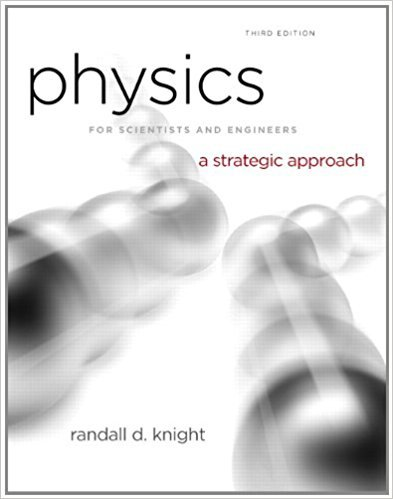×
Get Full Access to Physics For Scientists And Engineers: A Strategic Approach With Modern Physics - 3 Edition - Chapter 14 - Problem 27e
Get Full Access to Physics For Scientists And Engineers: A Strategic Approach With Modern Physics - 3 Edition - Chapter 14 - Problem 27e

×

# Sketch a position graph from t = 0 s to t = 10 s of aISBN: 9780321740908 69

## Solution for problem 27E Chapter 14

Physics for Scientists and Engineers: A Strategic Approach with Modern Physics | 3rd Edition

• Textbook Solutions
• 2901 Step-by-step solutions solved by professors and subject experts
• Get 24/7 help from StudySoup virtual teaching assistantsPhysics for Scientists and Engineers: A Strategic Approach with Modern Physics | 3rd Edition

4 5 1 407 Reviews
14
0
Problem 27E

Problem 27E

Sketch a position graph from t = 0 s to t = 10 s of a damped oscillator having a frequency of 1.0 Hz and a time constant of 4.0 s.

Step-by-Step Solution:

Step 1 of 2:

We are going to plot the position vs time graph for the given damped oscillator.

The frequency of the oscillation f = 1 Hz

The time constant 𝜏 = 4 s

The time range t = 0 to 10 s

Step 2 of 2

##### ISBN: 9780321740908

Physics for Scientists and Engineers: A Strategic Approach with Modern Physics was written by and is associated to the ISBN: 9780321740908. This textbook survival guide was created for the textbook: Physics for Scientists and Engineers: A Strategic Approach with Modern Physics, edition: 3. Since the solution to 27E from 14 chapter was answered, more than 325 students have viewed the full step-by-step answer. This full solution covers the following key subjects: constant, Damped, frequency, graph, having. This expansive textbook survival guide covers 17 chapters, and 1439 solutions. The answer to “Sketch a position graph from t = 0 s to t = 10 s of a damped oscillator having a frequency of 1.0 Hz and a time constant of 4.0 s.” is broken down into a number of easy to follow steps, and 31 words. The full step-by-step solution to problem: 27E from chapter: 14 was answered by , our top Physics solution expert on 08/30/17, 04:34AM.

Unlock Textbook Solution